# Length Converter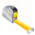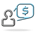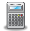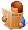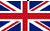Converter Currencies Calculator Articles

 Other units Area Energy Flowrate Force Length Mass Power Pressure Velocity Volume Temperature Time ______________ AcresAresBars Barrels per day Baryes BTUs BTUs per hour BTUs per minute BTUs per second Centimeters (cm) cm/sec Chains Cooking measures Crinals Cubic centimeters (cc) cc/sec cc-atmosphere Cubic decimeters Cubic feet Cubic feet per minute Cubic feet per second Cubic inches Cubic inches per minute Cubic inches per second Cubic meters Cubic meters per hour Cubic yards Cubic yards per minute Decimeters (dm) Drams DynesErgs per second Fluid drachmas Fluid ounces (fl.oz.) Feet (ft) Feet per hour Feet per minute Feet per second ft-lbf ft-lbf/hour ft-lbf/min ft-lbf/sec Furlongs Gallons Gallons per day Gallons per minute Gallons per second Gigawatts (GW) Gills Grains Grams (g) Gram-calories (g-cal) Hectares Hectometers Hundredweights (cwt) Inches (in) Inches H2O Inches Hg Inch-pound-forces (in-lbf) IST calories Joules (J) Joules per second (J/sec) Kilocalories (kcal) Kilograms (kg) kg-meters/sq. sec Kilogram-calories Kilogram-forces (kgf) Kgf/sq. cm Kgf/sq. meter Kilometers (km) Kilometers per hour (km/h) KiloPascal(kPa) Kilowatts (kW) Kilowatts per hour (kWh) Kips Knots Liters (l) Liters per minute Liters per second Long tons (imperial tons) MegaPascal(mPa) Megawatts (MW) Meters (m) Meters per minute Meters per second Metric horsepower Miles Miles per hour (mph) Miles per minute Milligrams (mg) Milliliters (ml) Millimeters (mm) Miner inches Newton (N) Ounces (oz) Ounce-force Pascal (Pa) Pints Pounds (lb) Pounds/sq. foot Pounds/sq. inch Poundals Poundals/sq. foot Poundals/sq. inch Pound-CHU Pound-force (lbf) Quarts Quarters Rods Shoe size Short tons/sq. footSlugs Square centimeters Square decimeters Square feet Square kilometers Square meters Square miles Square millimeters Square yards Stones Thermocalories TonsTons (refrigeration) Tonnes (metric tons) Torrs U.S. gallons U.S. horsepower per hour U.S. tons Watts Watts per hour Yards ________________ Ancient Greek: area Ancient Greek: currency Ancient Greek: length Ancient Greek: mass Ancient Greek: volume Ancient Roman: area Ancient Roman: length Ancient Roman: mass/coin Ancient Roman: volume Apothecary: imperial UK Apothecary: US/UK Apothecary: weightLeague units ________________ Calculator Contact Main website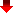Converting length units is an essential task in various fields such as engineering, construction, and design. It helps to ensure consistency and accuracy in measurements and allows for the comparison of dimensions between different systems.

In engineering, for instance, converting length units is crucial for the design of mechanical parts and the calculation of structural load capacity. In construction, converting length units helps to determine the length of materials required for building projects and ensures that the proper measurements are used for site preparation and excavation. In the field of design, converting length units is important for determining the size of objects in a project, such as furniture, doors, and windows, and for ensuring that they fit properly within the designated space.

Furthermore, converting length units helps to ensure the compatibility of products and systems from different countries, which is important for international trade and cooperation. Additionally, converting length units is important for accurately measuring distances, such as the length of a road or a bridge, which is essential for infrastructure planning and development. An example of this is converting miles to kilometers to determine the distance between two cities. Another example is converting inches to millimeters when designing a product, such as a device or machine, that requires precise measurements.

Finally, converting length units helps to avoid errors and miscommunications, which can have significant consequences in many fields.

MetricToImperial.com